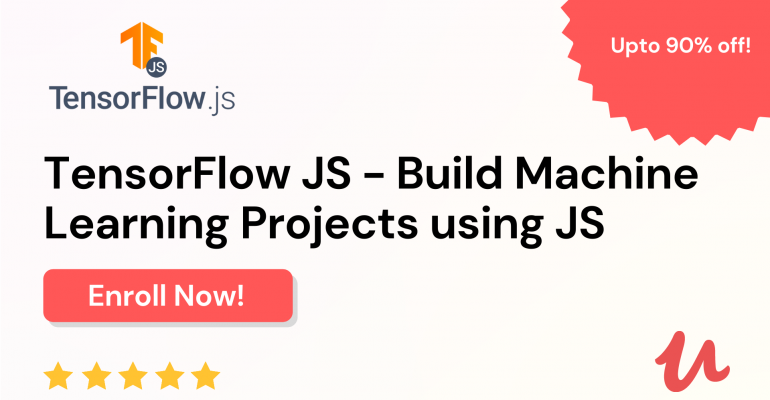Thanks to theidioms.com

## Best Course For Machine Learning With JavaScript – TensorFlow JS# Best Course For Machine Learning With JavaScript – TensorFlow JS

Are you looking to perform Machine Learning using JavaScript? Then, the ‘TensorFlow JS – Build Machine Learning Projects using JS’ course is a must for you!

Learn how to build Machine Learning projects using Javascript in this TensorFlow JS Course created by The Click Reader.

In this course, you will be learning about scalar as well as tensors and how to create them using TensorFlow.js. You will also be learning how to perform various kinds of tensor operations for manipulating and changing tensor values.

You will be performing a total of four Machine Learning projects while learning through this TensorFlow JS full course:

1. Linear Regression from Scratch

You will be learning how to create a Linear Regression model from scratch using TensorFlow.js. You will be preparing the data, building the model architecture as well as training the model using a custom-made loss function as well as an optimizer.

2. Linear Regression using a Sequential Model

You will be learning how to create a Linear Regression model using a Sequential Model with TensorFlow.js. You will be preparing the data, building the model architecture, training the model, viewing the change in the loss as well as build a multi-input regressor.

3. Logistic Regression using a Sequential Model

You will be learning how to create a Logistic Regression model using a Sequential Model with TensorFlow.js. You will be preparing the data, building the model architecture, training the model as well as build a deep neural network classifier.

4. Image Classification using MobileNet

You will be learning how to use a pre-trained model called MobileNet to build a dog breed classifier with TensorFlow.js.

By the end of this course, you will have learned how to build your own Machine Learning projects from scratch using TensorFlow JS.

Click here to enroll in TensorFlow JS – Build Machine Learning Projects using JS.

If you are interested in more things data, The Click Reader provides you a large collection of data science resources to study from—check it out by clicking here.